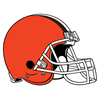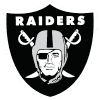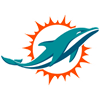DraftKings Make Playoffs Odds
-10000
0.01 to 1Patriots
90.8% implied probability

-5000
0.02 to 1Cowboys
93.6% implied probability

-5000
0.02 to 1Titans
92.7% implied probability

-2500
0.04 to 1Rams
91.4% implied probability

-1400
0.07 to 1Chiefs
88.2% implied probability

-1000
0.1 to 1Bills
87.2% implied probability

-500
0.2 to 1Ravens
78.9% implied probability

-280
0.36 to 1Chargers
70.5% implied probability

-190
0.53 to 149ers
61.6% implied probability

-110
0.91 to 1Bengals
50.0% implied probability

+100
1 to 1Colts
47.8% implied probability

+125
1.25 to 1Eagles
41.7% implied probability

+125
1.25 to 1Football Team
42.2% implied probability

+250
2.5 to 1Browns
26.7% implied probability

+250
2.5 to 1Vikings
26.7% implied probability

+350
3.5 to 1Steelers
21.1% implied probability

+350
3.5 to 1Broncos
21.1% implied probability

+350
3.5 to 1Saints
21.1% implied probability

+650
6.5 to 1Raiders
12.6% implied probability

+800
8 to 1Falcons
10.4% implied probability

+800
8 to 1Dolphins
10.6% implied probability

+1000
10 to 1Seahawks
8.6% implied probability

+2500
25 to 1Giants
3.7% implied probability

+5000
50 to 1Bears
% implied probability

+100000
1000 to 1Jets
% implied probability

+100000
1000 to 1Lions
% implied probability

+100000
1000 to 1Jaguars
% implied probability